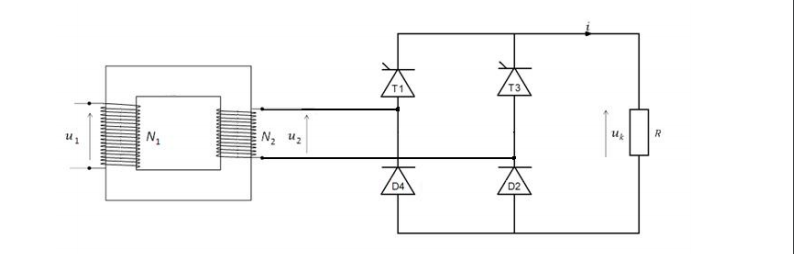# Rectifier and thyristor

I'm wondering as to how a system like this:acts, when their thyristors are controlled in various ways.

The converters are simple enough, but I'm wondering how the graph made around the voltage going over the resistor $R$ would look like when the thyristors are set top $0^\circ$ and $90^\circ$ respectively.

• On-line simulator: plexim.com/academy/power-electronics/thyristor-rect-ind-load – Enric Blanco Apr 19 '17 at 10:41
• Thanks, that was a massive help. How does the frequency of the current project to the frequency of the sine-waves; is it directly relatable? (50 hz would mean that one complete sine-wave or two converted "mounds" are 20 milliseconds?) – Grak Apr 19 '17 at 10:52

## 1 Answer

There are several alternative topologies for controlled rectifier bridges. The one in your diagram is called a half-controlled bridge. The associated waveform is shown below.Here is the description of operation:The above material is from:F. F. Mazda, Thyristor Control, John Wiley & Sons,1973

• it would definitely be helpful to include actual text rather than an image of text. – NH. Dec 11 '17 at 16:01